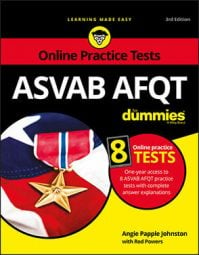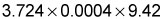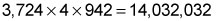##### ASVAB AFQT For DummiesYou are likely to encounter a question on an ASVAB AFQT math subtest that asks you to multiply decimals together. The best way to get the right answer is through the following three-step process:
1. Convert the decimals to whole numbers by moving the decimal points to the right, remembering to count how many spaces you move each decimal point.
2. Multiply the whole numbers just as you'd perform any other multiplication.
3. Place the decimal point in the product by moving the decimal point to the left the same number of total spaces you moved the decimal points to the right at the beginning.
Let's try an example: multiplyFirst, convert the decimals to whole numbers by moving the decimal points to the right (remember to count).

• 3.724 becomes 3,724 (decimal moved three spaces).
• 0.0004 becomes 4 (decimal moved four spaces).
• 9.42 becomes 942 (decimal moved two spaces).
Second, perform the multiplication on the whole numbers:For the third step, put the decimal point in the correct position by moving it to the left the same number of places you moved the points to the right. You moved the decimal points a total of nine spaces to the right at the beginning, so now place the decimal point nine spaces to the left:

14,032,032 becomes 0.014032032

If you run out of numbers before you're finished counting spaces to the left, add zeros (as shown in the example) until you've finished counting.